# A solid metal sphere of radius`
Question:

A solid metal sphere of radius $R$ having charge $q$ is enclosed inside the concentric spherical shell of inner radius $a$ and outer radius $b$ as shown in figure. The approximate variation electric field $\overrightarrow{\mathrm{E}}$ as a function of distance $r$ from centre $O$ is given by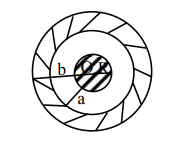1.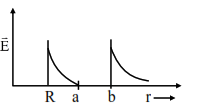2.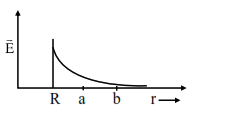3.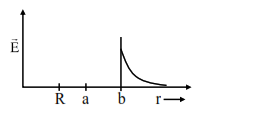4.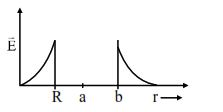Correct Option: 1

Solution:

Considering outer spherical shell is nonconducting

Electric field inside a metal sphere is zero.

$\mathrm{r}<\mathrm{R} \Rightarrow \mathrm{E}=0$

$r>R \Rightarrow E=\frac{k Q}{r^{2}}$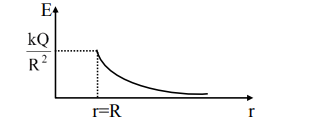Option (2)

Considering outer spherical shell is conducting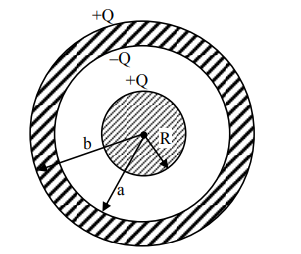$\mathrm{r}<\mathrm{R}, \mathrm{E}=0$

$\mathrm{R} \leq \mathrm{r}<\mathrm{a}$

$\mathrm{E}=\frac{\mathrm{kQ}}{\mathrm{r}^{2}}$

$a \leq r$\mathrm{E}=0r \geq b\mathrm{E}=\frac{\mathrm{kQ}}{\mathrm{r}^{2}}\$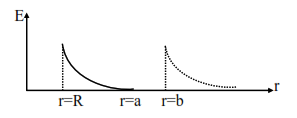Option (1)